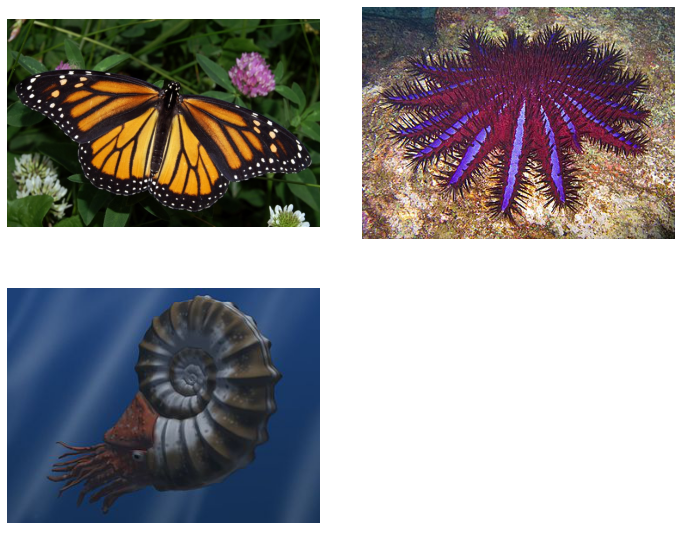Betul Mescioglu

# Edge Detection:

DESCRIPTION

Detecting changes in intensity for the purpose of finding edges can be accomplished using first- or second-order derivatives. To find the edge strength and direction at an arbitrary location of an image, f, the gradient of f is used. This vector points in the direction of maximum rate of change of f at a certain point. To obtain the gradient of an image, we need to take the partial derivatives along x and y axis for each pixel. Since digitized images are discrete and made out of pixels, we need to use kernels to achieve this. 1-D kernels [-1,1] and transpose([-1, 1]) can be convolved with image pixels to find the derivatives in x and y direction. If we want to detect edges in the diagonal direction, we can use 2-D kernels. This time diagonal differences are calculated. Three operators are discussed in this project:

• Roberts: 2-D kernels with [[-1, 0],[0,1]] for x-axis and [[0,-1],[1,0]] for y-axis are passed through the image.
• Prewitt: 2-D kernels with [[-1,-1,-1],[0,0,0],[1,1,1]] for x-axis and [[-1,0,1],[-1,0,1],[-1,0,1]] for y-axis are passed through the image
• Sobel: 2-D kernels with [[-1,-2,-1],[0,0,0],[1,2,1]] for x-axis and [[-1,0,1],[-2,0,2],[-1,0,1]] for y-axis are passed through the image.

ALGORITHM

Any of the pairs of kernels are convolved with the image to obtain the gradient components gx and gy at every pixel location. That is, we obtain groups of pixels from the image with the same size as the kernel, get the dot product of this sample and the kernel and add the numbers. This process is done for both kernels producing gx and gy. To calculate the edge strength we need to find the magnitude of the gradient which is the square root(sum(gx squared and gy squared)). (To find the direction of the gradient vector we find arctan(gy/gx) if necessary.) Finally, this magnitude is assigned to all three channels of the corresponding pixel in the produced image.

``````import skimage.io
import numpy as np
import matplotlib.pyplot as plt

#importing images:
fig, ax = plt.subplots(ncols=2,nrows=2, figsize=(10,8))
ax = ax.ravel()
fig.tight_layout()
#Plot the original images
ax.imshow(butterfly)
ax.imshow(thorns)
ax.imshow(spiral)
for a in ax:
a.axis('off')

``````FILTER FUNCTIONS

``````#sobel
def sobel(img):
#define the vertical filter
vertical_filter = [[-1,-2,-1], [0,0,0], [1,2,1]]

#define the horizontal filter
horizontal_filter = [[-1,0,1], [-2,0,2], [-1,0,1]]

#get the dimensions of the image
n,m,d = img.shape

#initialize the edges image
edges_img = img.copy()

#loop over all pixels in the image
for row in range(3, n-2):
for col in range(3, m-2):

#create little local 3x3 box
local_pixels = img[row-1:row+2, col-1:col+2, 0]

#apply the vertical filter
vertical_transformed_pixels = vertical_filter*local_pixels
#remap the vertical score
#print(vertical_transformed_pixels)
vertical_score = vertical_transformed_pixels.sum()/4
#apply the horizontal filter
#print(vertical_score)
horizontal_transformed_pixels = horizontal_filter*local_pixels
#remap the horizontal score
horizontal_score = horizontal_transformed_pixels.sum()/4

#combine the horizontal and vertical scores into a total edge score
edge_score = (vertical_score**2 + horizontal_score**2)**.5

#insert this edge score into the edges image
edges_img[row, col] = [edge_score]*3
#remap the values in the 0-1 range in case they went out of bounds
edges_img = edges_img/edges_img.max()
return edges_img``````
``````#Roberts
def roberts(img):
#define the vertical filter
vertical_filter = [[-1,0], [0,1]]

#define the horizontal filter
horizontal_filter = [[0,-1], [1,0]]

#get the dimensions of the image
n,m,d = img.shape

#initialize the edges image
edges_img = img.copy()

#loop over all pixels in the image
for row in range(3, n-2):
for col in range(3, m-2):

#create little local 3x3 box
local_pixels = img[row-1:row+1, col-1:col+1, 0]

#apply the vertical filter
vertical_transformed_pixels = vertical_filter*local_pixels
#remap the vertical score
vertical_score = vertical_transformed_pixels.sum()/4

#apply the horizontal filter
horizontal_transformed_pixels = horizontal_filter*local_pixels
#remap the horizontal score
horizontal_score = horizontal_transformed_pixels.sum()/4

#combine the horizontal and vertical scores into a total edge score
edge_score = (vertical_score**2 + horizontal_score**2)**.5

#insert this edge score into the edges image
edges_img[row, col] = [edge_score]*3

#remap the values in the 0-1 range in case they went out of bounds
edges_img = edges_img/edges_img.max()
return edges_img``````
``````#Prewitt
def prewitt(img):
#define the vertical filter
vertical_filter = [[-1,-1,-1], [0,0,0], [1,1,1]]

#define the horizontal filter
horizontal_filter = [[-1,0,1], [-1,0,1], [-1,0,1]]

#get the dimensions of the image
n,m,d = img.shape

#initialize the edges image
edges_img = img.copy()

#loop over all pixels in the image
for row in range(3, n-2):
for col in range(3, m-2):

#create little local 3x3 box
local_pixels = img[row-1:row+2, col-1:col+2, 0]

#apply the vertical filter
vertical_transformed_pixels = vertical_filter*local_pixels
#remap the vertical score
vertical_score = vertical_transformed_pixels.sum()/4

#apply the horizontal filter
horizontal_transformed_pixels = horizontal_filter*local_pixels
#remap the horizontal score
horizontal_score = horizontal_transformed_pixels.sum()/4

#combine the horizontal and vertical scores into a total edge score
edge_score = (vertical_score**2 + horizontal_score**2)**.5

#insert this edge score into the edges image
edges_img[row, col] = [edge_score]*3

#remap the values in the 0-1 range in case they went out of bounds
edges_img = edges_img/edges_img.max()
return edges_img``````

RESULTS

``````fig, ax = plt.subplots(ncols=2,nrows=2, figsize=(10,8), sharex=True, sharey=True)
ax = ax.ravel()
fig.tight_layout()
#Plot the original image
ax.imshow(butterfly)
ax.set_title('Original Image')
#Plot the Sobel filter applied image
ax.imshow(sobel(butterfly))
ax.set_title('Sobel Filter Applied Image')
#Plot the Prewitt filter applied image
ax.imshow(prewitt(butterfly))
ax.set_title('Prewitt Filter Applied Image')
#Plot the Roberts filter applied image
ax.imshow(roberts(butterfly))
ax.set_title('Roberts Filter Applied Image')
for a in ax:
a.axis('off')

``````